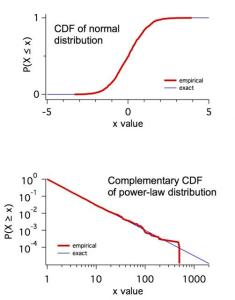# Cumulative Distribution Function (CDF)

The code below will estimate the cumulative distribution function, P(X≥x) from a wave of observations. There is an option to plot the complementary cumulative distribution function, P(X≤x), as well. Note that an alternative way to calculate this would be to create an ordinary histogram and integrate. The version presented here is without binning. The two advantages are that (1) you don't have to worry about optimal bin size and (2) you do not lose any information.

The attached example program shows typical results.

Function MakeCDF(w,sortflag)                // creates empirical cumulative distribution function
wave w; variable sortflag
variable n=numpnts(w)
string wroot=NameofWave(w),cdfname=wroot+"_cdf"
duplicate /o w \$cdfname
wave cdfwave=\$cdfname

if (sortflag == 1)
Sort /R cdfwave cdfwave             // largest values first (for complementary CDF)
else
Sort cdfwave cdfwave                // smallest values first (for CDF);  default case
endif

SetScale x 0,1,"", cdfwave              // fraction of x values
EndForumSupportGallery